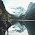## Group Problem:

1.) If there's 6 girls per 2 boys, how many girls are there if there's only one boy?

2.) If there's 2 papers per pencil, how many papers are there if there's 10 pencils?

Own Problem:

1.)There are 2 cookies per milk, how many cookies are there if there's 3 milks?

2.)If there's 10 chairs per 5 tables, how many chairs are there if there's one table?

Video:

5:28 PM

#### 4 comments :

1.#2 in 1 table theirs 2 chairs 10/5=2

2.This comment has been removed by the author.

3.If there's 15 chairs per 3 tables, how many chairs are there if there's one table?

1.The answer for your question is : There would be 5 chairs per table.